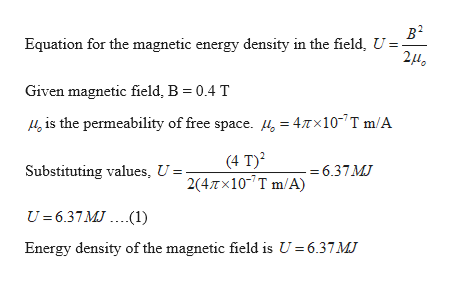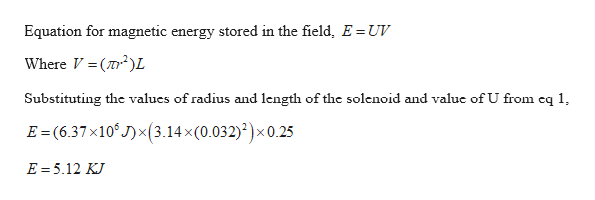# The magnetic field inside a superconducting solenoid is 4.0 T.The solenoid has an inner radius of 3.2 cm and a length of 25.0 cm.Determine (a) the magnetic energy density in the field and (b) the energystored in the magnetic field within the solenoid.diagram must be inclded if needed please

Question

The magnetic field inside a superconducting solenoid is 4.0 T.
The solenoid has an inner radius of 3.2 cm and a length of 25.0 cm.
Determine (a) the magnetic energy density in the field and (b) the energy
stored in the magnetic field within the solenoid.

diagram must be inclded if needed please

check_circleExpert Solution
Step 1

Given:

Magnetic field inside a solenoid = 4 T

Inner radius of the solenoid, r = 3.2 cm = 0.032 m

Length of the solenoid, L = 25 cm = 0.25 m

Step 2

Calculating the magnetic energy density in the field:help_outlineImage Transcriptioncloseв2 Equation for the magnetic energy density in the field, U =- 2HO Given magnetic field, B = 0.4 T is the permeability of free space. 4, = 47Tx10 T m/A (4 T)2 2(4Tx107 T m/A) Substituting values, U 6.37MJ U= 6.37MJ.(1) Energy density of the magnetic field is U 6.37 MJ fullscreen
Step 3

Calculating the magnetic energy stored in th...help_outlineImage TranscriptioncloseEquation for magnetic energy stored in the field, E = UV Where V (2L Substituting the values of radius and length of the solenoid and value of U from eq 1, E (6.37x10 J)(3.14x(0.032))x 0.25 E 5.12 KJ fullscreen

### Want to see the full answer?

See Solution

#### Want to see this answer and more?

Solutions are written by subject experts who are available 24/7. Questions are typically answered within 1 hour*

See Solution
*Response times may vary by subject and question
Tagged in

### Physics